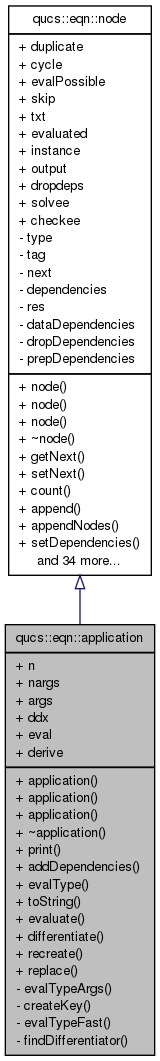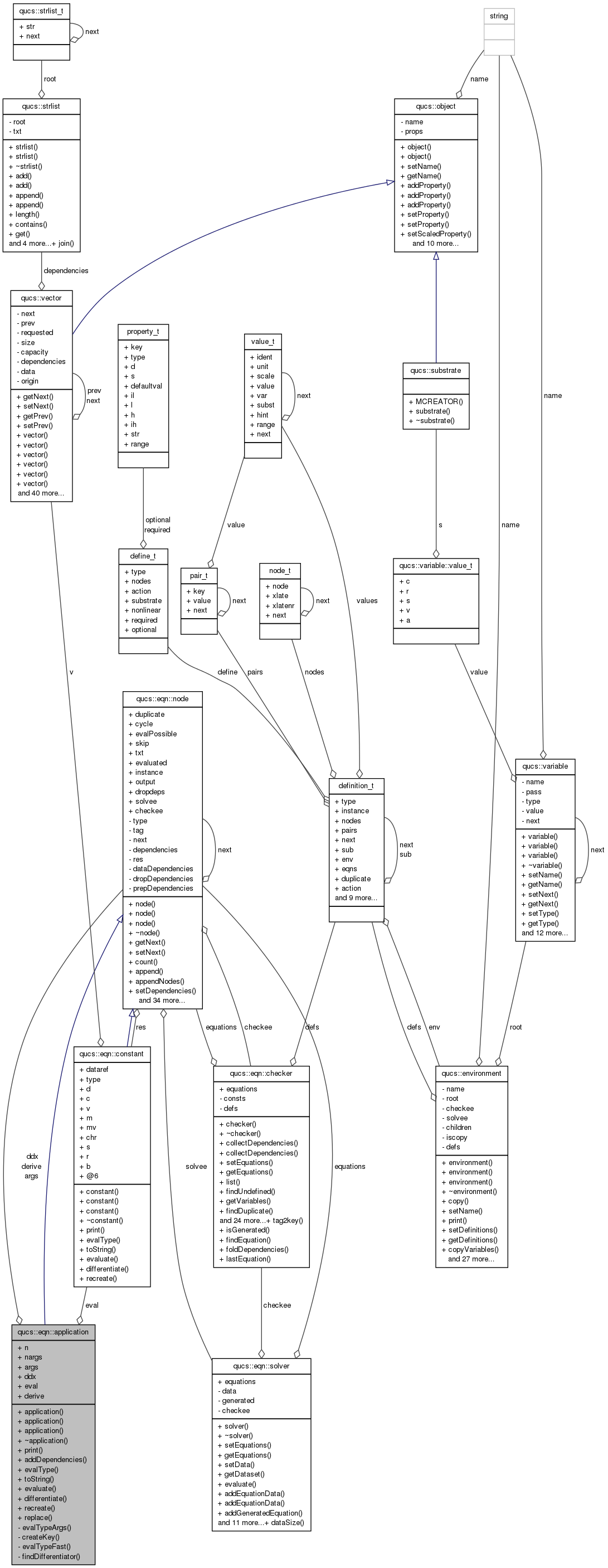Qucs-core  0.0.19
qucs::eqn::application Class Reference

`#include <equation.h>`

Inheritance diagram for qucs::eqn::application:[legend]
Collaboration diagram for qucs::eqn::application:[legend]

## Public Member Functions

application ()
application (const application &)
application (const char *, int)
~application ()
void print (void)
void addDependencies (strlist *)
int evalType (void)
char * toString (void)
constantevaluate (void)
nodedifferentiate (char *)
noderecreate (void)
void replace (char *, char *)

## Data Fields

char * n
int nargs
nodeargs
nodeddx
evaluator_t eval
differentiator_t derive

## Private Member Functions

void evalTypeArgs (void)
char * createKey (void)
int evalTypeFast (void)
int findDifferentiator (void)

## Detailed Description

Definition at line 237 of file equation.h.

## Constructor & Destructor Documentation

 qucs::application::application ( )

Definition at line 592 of file equation.cpp.

 qucs::application::application ( const application & o )

Definition at line 616 of file equation.cpp.

 qucs::application::application ( const char * func, int a )

Definition at line 604 of file equation.cpp.

 qucs::application::~application ( )

Definition at line 652 of file equation.cpp.

## Member Function Documentation

 void qucs::application::addDependencies ( strlist * depends ) ` [virtual]`

Reimplemented from qucs::eqn::node.

Definition at line 756 of file equation.cpp.

 char * qucs::application::createKey ( void ) ` [private]`

Definition at line 790 of file equation.cpp.

 node * qucs::application::differentiate ( char * derivative ) ` [virtual]`

Reimplemented from qucs::eqn::node.

Definition at line 988 of file equation.cpp.

 int qucs::application::evalType ( void ) ` [virtual]`

Reimplemented from qucs::eqn::node.

Definition at line 827 of file equation.cpp.

 void qucs::application::evalTypeArgs ( void ) ` [private]`

Definition at line 767 of file equation.cpp.

 int qucs::application::evalTypeFast ( void ) ` [private]`

Definition at line 804 of file equation.cpp.

 constant * qucs::application::evaluate ( void ) ` [virtual]`

Reimplemented from qucs::eqn::node.

Definition at line 908 of file equation.cpp.

 int qucs::application::findDifferentiator ( void ) ` [private]`

Definition at line 892 of file equation.cpp.

 void qucs::application::print ( void ) ` [virtual]`

Reimplemented from qucs::eqn::node.

Definition at line 666 of file equation.cpp.

 node * qucs::application::recreate ( void ) ` [virtual]`

Reimplemented from qucs::eqn::node.

Definition at line 636 of file equation.cpp.

 void qucs::application::replace ( char * src, char * dst ) ` [virtual]`

Reimplemented from qucs::eqn::node.

Definition at line 642 of file equation.cpp.

 char * qucs::application::toString ( void ) ` [virtual]`

Reimplemented from qucs::eqn::node.

Definition at line 672 of file equation.cpp.

## Field Documentation

Definition at line 256 of file equation.h.

Definition at line 257 of file equation.h.

Definition at line 259 of file equation.h.

Definition at line 258 of file equation.h.

Definition at line 254 of file equation.h.

Definition at line 255 of file equation.h.

The documentation for this class was generated from the following files: